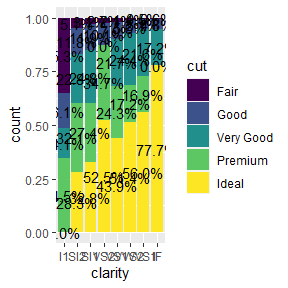# Compute custom proportions with stat_prop()

library(ggstats)
library(ggplot2)

stat_prop() is a variation of ggplot2::stat_count() allowing to compute custom proportions according to the by aesthetic defining the denominator (i.e. all proportions for a same value of by will sum to 1). The by aesthetic should be a factor. Therefore, stat_prop() requires the by aesthetic and this by aesthetic should be a factor.

## Adding labels on a percent stacked bar plot

When using position = "fill" with geom_bar(), you can produce a percent stacked bar plot. However, the proportions corresponding to the y axis are not directly accessible using only ggplot2. With stat_prop(), you can easily add them on the plot.

In the following example, we indicated stat = "prop" to ggplot2::geom_text() to use stat_prop(), we defined the by aesthetic (here we want to compute the proportions separately for each value of x), and we also used ggplot2::position_fill() when calling ggplot2::geom_text().

d <- as.data.frame(Titanic)
p <- ggplot(d) +
aes(x = Class, fill = Survived, weight = Freq, by = Class) +
geom_bar(position = "fill") +
geom_text(stat = "prop", position = position_fill(.5))
p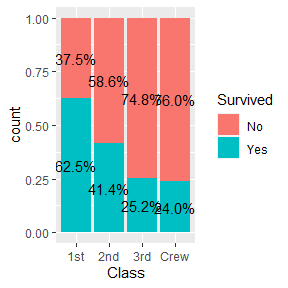Note that stat_prop() has properly taken into account the weight aesthetic.

stat_prop() is also compatible with faceting. In that case, proportions are computed separately in each facet.

p + facet_grid(cols = vars(Sex))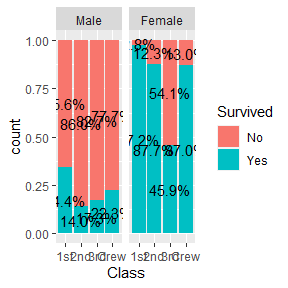## Displaying proportions of the total

If you want to display proportions of the total, simply map the by aesthetic to 1. Here an example using a stacked bar chart.

ggplot(d) +
aes(x = Class, fill = Survived, weight = Freq, by = 1) +
geom_bar() +
geom_text(
aes(label = scales::percent(after_stat(prop), accuracy = 1)),
stat = "prop",
position = position_stack(.5)
)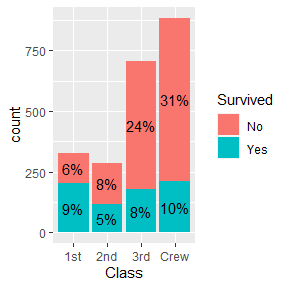## A dodged bar plot to compare two distributions

A dodged bar plot could be used to compare two distributions.

ggplot(d) +
aes(x = Class, fill = Sex, weight = Freq, by = Sex) +
geom_bar(position = "dodge")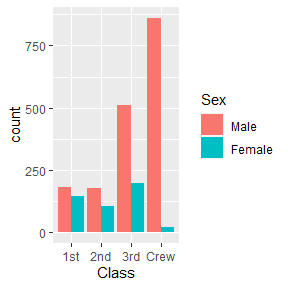On the previous graph, it is difficult to see if first class is over- or under-represented among women, due to the fact they were much more men on the boat. stat_prop() could be used to adjust the graph by displaying instead the proportion within each category (i.e. here the proportion by sex).

ggplot(d) +
aes(x = Class, fill = Sex, weight = Freq, by = Sex, y = after_stat(prop)) +
geom_bar(stat = "prop", position = "dodge") +
scale_y_continuous(labels = scales::percent)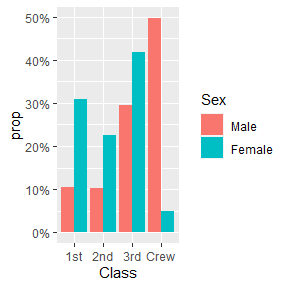The same example with labels:

ggplot(d) +
aes(x = Class, fill = Sex, weight = Freq, by = Sex, y = after_stat(prop)) +
geom_bar(stat = "prop", position = "dodge") +
scale_y_continuous(labels = scales::percent) +
geom_text(
mapping = aes(
label = scales::percent(after_stat(prop), accuracy = .1),
y = after_stat(0.01)
),
vjust = "bottom",
position = position_dodge(.9),
stat = "prop"
)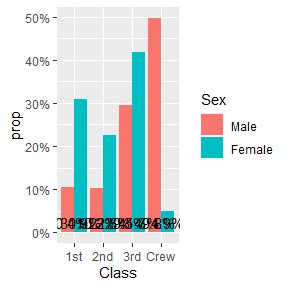## Displaying unobserved levels

With the complete argument, it is possible to indicate an aesthetic for those statistics should be completed for unobserved values.

d <- diamonds %>%
dplyr::filter(!(cut == "Ideal" & clarity == "I1")) %>%
dplyr::filter(!(cut == "Very Good" & clarity == "VS2")) %>%
dplyr::filter(!(cut == "Premium" & clarity == "IF"))
p <- ggplot(d) +
aes(x = clarity, fill = cut, by = clarity) +
geom_bar(position = "fill")
p +
geom_text(
stat = "prop",
position = position_fill(.5)
)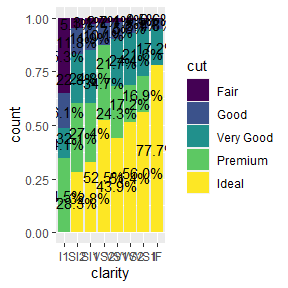Adding complete = "fill" will generate “0.0%” labels where relevant.

p +
geom_text(
stat = "prop",
position = position_fill(.5),
complete = "fill"
)